# Problem Solving Using Linear Regression: Steps & Examples

An error occurred trying to load this video.

Try refreshing the page, or contact customer support.

Coming up next: Analyzing Residuals: Process & Examples

### You're on a roll. Keep up the good work!

Replay
Your next lesson will play in 10 seconds
• 0:00 Linear Regression Scenario
• 0:25 Key Terms
• 2:18 Using Linear Regression
• 4:37 Finding 'A'
• 5:38 Finding 'B' & Graphing
• 7:08 Lesson Summary

Want to watch this again later?

Timeline
Autoplay
Autoplay
Speed

#### Recommended Lessons and Courses for You

Lesson Transcript
Instructor: Cathryn Jackson

Cat has taught a variety of subjects, including communications, mathematics, and technology. Cat has a master's degree in education and is currently working on her Ph.D.

Linear regression can be a powerful tool for predicting and interpreting information. Learn to use two common formulas for linear regression in this lesson.

## Linear Regression Scenario

Jake has decided to start a hot dog business. He has hired his cousin, Noah, to help him with hot dog sales. But there's a problem! Noah can only work 20 hours a week. Jake wants to have Noah working at peak hot dog sales hours. How can he find this information? In this lesson, you will learn how to solve problems using concepts based on linear regression. First, let's check out some of our key terms that will be beneficial in this lesson.

## Key Terms

Jake will have to collect data and use regression analysis to find the optimum hot dog sale time. Regression analysis is the study of two variables in an attempt to find a relationship, or correlation. For example, there have been many regression analyses on student study hours and GPA. Studies have found a relationship between the number of hours a student studies and their overall GPA.

In other words, the number of hours a student studies is the independent variable and the GPA is the dependent variable. The student's GPA will depend on the number of hours a student studies; therefore, there is a relationship between the two variables. We'll talk more about this relationship, also known as correlation, in a minute, but let's define linear regression next.

A regression line is a straight line that attempts to predict the relationship between two points, also known as a trend line or line of best fit. You've probably seen this line previously in another class. Linear regression is a prediction when a variable (y) is dependent on a second variable (x) based on the regression equation of a given set of data.

To clarify, you can take a set of data, create a scatter plot, create a regression line, and then use regression analysis to see if you have a correlation. Once you have your correlation, you have linear regression. Okay, that probably sounded like Greek to you. Let's talk a little bit about correlation before looking at some examples.

A correlation is the relationship between two sets of variables used to describe or predict information. The stronger the relationship between the two sets of variables, the more likely your prediction will be accurate. We will examine this concept of correlation more closely in other lessons, such as Interpreting Linear Relationships Using Data and Correlation Versus Causation. For now, let's focus on using the regression line to help solve Jake's hot dog sales dilemma.

## Using Linear Regression

First, let's look at the data for Jake's hot dog sales. Jake has been working for the past few weeks from 1 pm to 7 pm each day. Each day, Jake has tracked the hour and the number of hot dog sales for each hour. Take a look at this data set for Monday:

(1, 10) (2, 11) (3, 15) (4, 12) (5, 17) (6, 18) (7, 20)

To establish the relationship between the time of day and the number of hot dogs sold, Jake will need to put the data into the formula y = ax + b. You've probably seen the formula for slope intercept form in algebra: y = mx + b. This is the same formula, but in statistics, we've replaced the m with a; a is still slope in this formula, so there aren't any big changes you need to worry about.

To find the regression line for this data set, let's first put this information into a chart like this: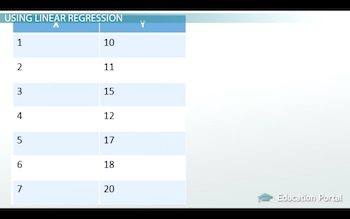Now we need to use the least squares formula to find our variables in y = ax + b. This is the formula to find the slope a: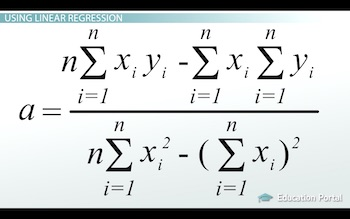I know, it looks pretty complicated. First, we will need a little more information in our chart. Look at how I expanded the chart to include x times y and x squared: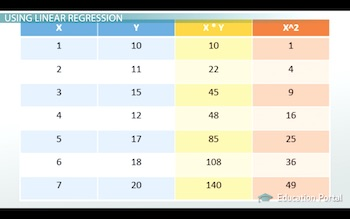Look at the first row in this chart. In the first column I have 1, my value for the first x in the data set. In the second column, I have 10, my value for the first y in the data set. Under x times y, I have 10, which is the product of the first and second column. In the last column, under x squared, I have 1, which is the value of the first column squared. Notice that each row on this chart follows this same pattern.

Now, we've added a final row that shows the sum of each column: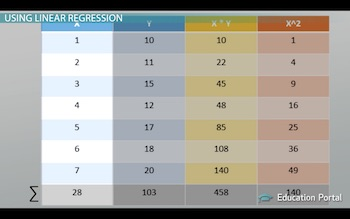For example, the first column has all of the x values: 1, 2, 3, 4, 5, 6, 7. The last row is the sum of all of those values: 1 + 2 + 3 + 4 + 5 + 6 + 7 = 28. So, let's review our chart; we have:

1. All of the x values
2. All of the y values
3. x * y for each ordered pair
4. x^2 for each x value
5. The sum of x, y, x * y, and x^2

## Finding A

Now that we have this information, let's look at our formula and our chart. I've circled the places in our formula with the corresponding values in our chart with similarly colored circles: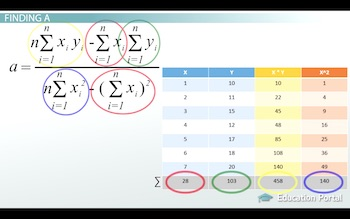To unlock this lesson you must be a Study.com Member.

### Register to view this lesson

Are you a student or a teacher?

### Unlock Your Education

#### See for yourself why 30 million people use Study.com

##### Become a Study.com member and start learning now.
Back
What teachers are saying about Study.com

### Earning College Credit

Did you know… We have over 160 college courses that prepare you to earn credit by exam that is accepted by over 1,500 colleges and universities. You can test out of the first two years of college and save thousands off your degree. Anyone can earn credit-by-exam regardless of age or education level.